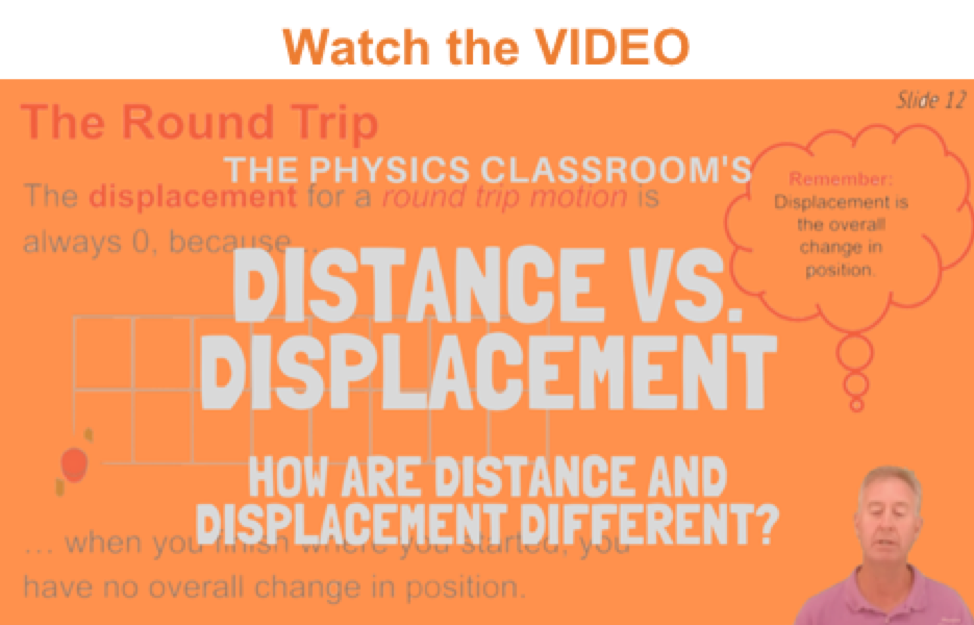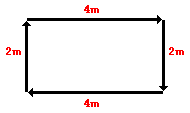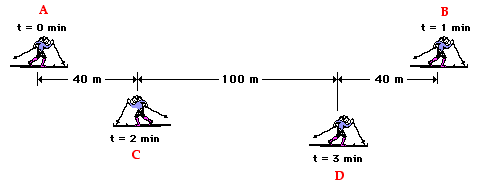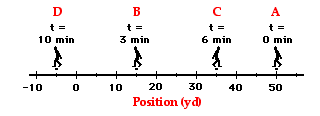1-D Kinematics - Lesson 1 - Describing Motion with Words

# Distance and DisplacementDistance and displacement are two quantities that may seem to mean the same thing yet have distinctly different definitions and meanings.

• Distance is a scalar quantity that refers to "how much ground an object has covered" during its motion.
• Displacement is a vector quantity that refers to "how far out of place an object is"; it is the object's overall change in position.

To test your understanding of this distinction, consider the motion depicted in the diagram below. A physics teacher walks 4 meters East, 2 meters South, 4 meters West, and finally 2 meters North.Even though the physics teacher has walked a total distance of 12 meters, her displacement is 0 meters. During the course of her motion, she has "covered 12 meters of ground" (distance = 12 m). Yet when she is finished walking, she is not "out of place" - i.e., there is no displacement for her motion (displacement = 0 m). Displacement, being a vector quantity, must give attention to direction. The 4 meters east cancels the 4 meters west; and the 2 meters south cancels the 2 meters north. Vector quantities such as displacement are direction aware. Scalar quantities such as distance are ignorant of direction. In determining the overall distance traveled by the physics teachers, the various directions of motion can be ignored.

Now consider another example. The diagram below shows the position of a cross-country skier at various times. At each of the indicated times, the skier turns around and reverses the direction of travel. In other words, the skier moves from A to B to C to D.Use the diagram to determine the resulting displacement and the distance traveled by the skier during these three minutes. Then click the button to see the answer.As a final example, consider a football coach pacing back and forth along the sidelines. The diagram below shows several of coach's positions at various times. At each marked position, the coach makes a "U-turn" and moves in the opposite direction. In other words, the coach moves from position A to B to C to D.What is the coach's resulting displacement and distance of travel? Click the button to see the answer.To understand the distinction between distance and displacement, you must know the definitions. You must also know that a vector quantity such as displacement is direction-aware and a scalar quantity such as distance is ignorant of direction. When an object changes its direction of motion, displacement takes this direction change into account; heading the opposite direction effectively begins to cancel whatever displacement there once was.

### Check Your Understanding

1. What is the displacement of the cross-country team if they begin at the school, run 10 miles and finish back at the school?

2. What is the distance and the displacement of the race car drivers in the Indy 500?

Next Section: